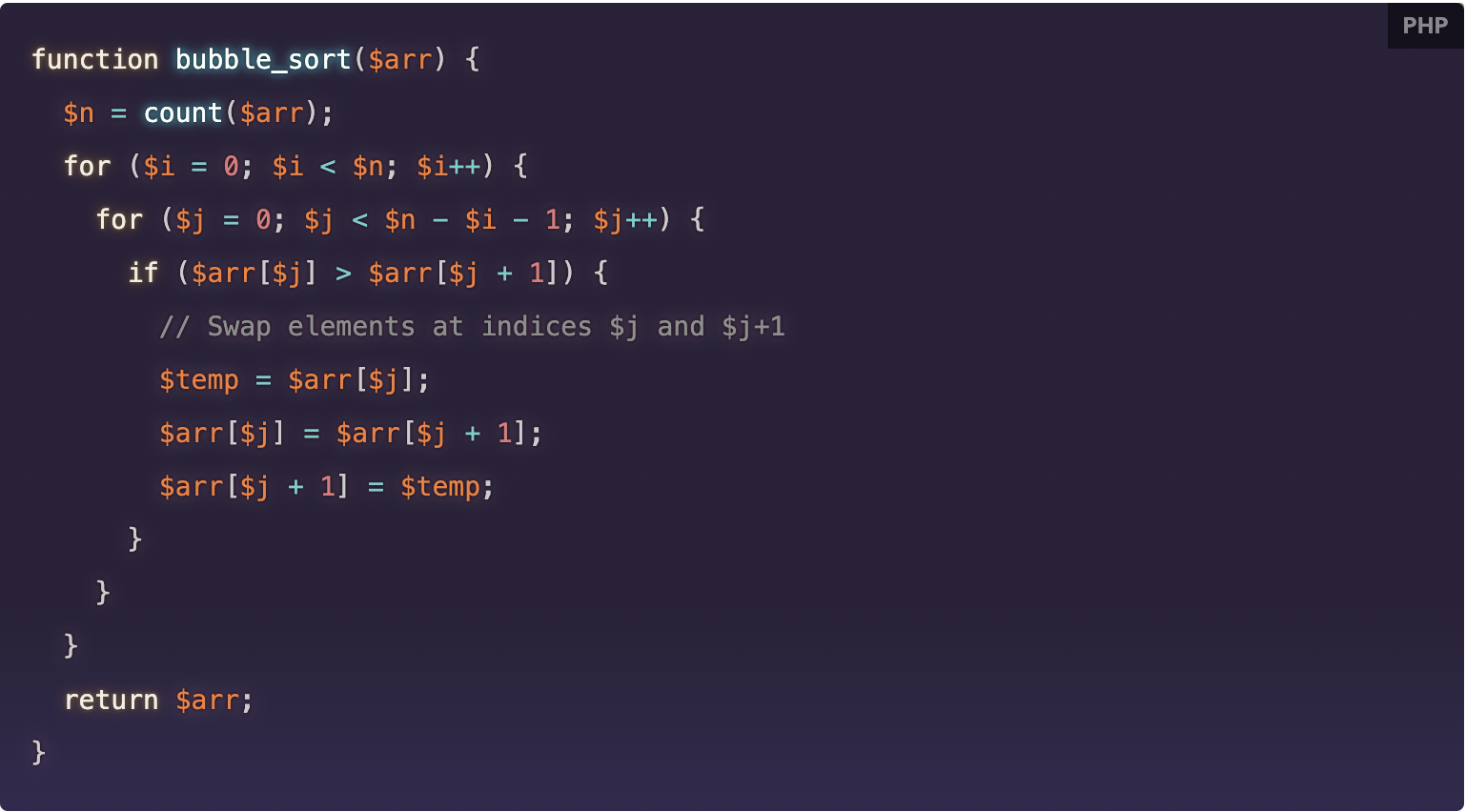# How to implement the bubble sort in PHP

What is bubble sort?

Bubble sort is a simple sorting algorithm that repeatedly iterates through an array, comparing adjacent elements and swapping them if they are in the wrong order. It is called "bubble sort" because the larger elements "bubble" to the top of the array as they are being sorted.## How does bubble sort work?

The basic idea of bubble sort is to iterate through the array, comparing adjacent elements and swapping them if necessary. This process is repeated until the entire array is sorted.

Here is an example of how bubble sort works on an array of integers:

``[5, 3, 8, 2, 1, 4]``

1. The first pass of the bubble sort algorithm compares the first two elements, `5` and `3`. Since `3` is smaller than `5`, they are swapped:

``[3, 5, 8, 2, 1, 4]``

The next pass compares the next two elements, `5` and `8`. Since they are already in the correct order, they are not swapped.

The third pass compares the next two elements, `8` and `2`. Since `2` is smaller than `8`, they are swapped:

``[3, 5, 2, 8, 1, 4]``

The fourth pass compares the next two elements, `8` and `1`. Since `1` is smaller than `8`, they are swapped:

``[3, 5, 2, 1, 8, 4]``

The fifth pass compares the final two elements, `8` and `4`. Since `4` is smaller than `8`, they are swapped:

``[3, 5, 2, 1, 4, 8]``

At this point, the array is not fully sorted, so the bubble sort algorithm repeats the process, starting at the beginning of the array again.

The sixth pass compares the first two elements, `3` and `5`. Since they are already in the correct order, they are not swapped.

The seventh pass compares the next two elements, `5` and `2`. Since `2` is smaller than `5`, they are swapped:

``[3, 2, 5, 1, 4, 8]``

The eighth pass compares the next two elements, `5` and `1`. Since `1` is smaller than `5`, they are swapped:

``[3, 2, 1, 5, 4, 8]``

The ninth pass compares the next two elements, `5` and `4`. Since `4` is smaller than `5`, they are swapped:

``[3, 2, 1, 4, 5, 8]``

The tenth pass compares the final two elements, `5` and `8`. Since `8` is larger than `5`, they are not swapped. At this point, the array is fully sorted, and the bubble sort algorithm ends. The final sorted array is:

``[3, 2, 1, 4, 5, 8]``

## How to implement bubble sort in

``````function bubble_sort(\$arr) {
\$n = count(\$arr);
for (\$i = 0; \$i < \$n; \$i++) {
for (\$j = 0; \$j < \$n - \$i - 1; \$j++) {
if (\$arr[\$j] > \$arr[\$j + 1]) {
// Swap elements at indices \$j and \$j+1
\$temp = \$arr[\$j];
\$arr[\$j] = \$arr[\$j + 1];
\$arr[\$j + 1] = \$temp;
}
}
}
return \$arr;
}``````

This function takes an array as input and returns a new array with the elements sorted in ascending order. The basic idea of bubble sort is to repeatedly iterate through the array, comparing adjacent elements and swapping them if they are in the wrong order. The inner loop starts at the beginning of the array and compares each pair of adjacent elements, swapping them if necessary. The outer loop then repeats this process for the next element, until the entire array is sorted.

Here is an example of how you can use this function:

``````\$arr = [5, 3, 8, 2, 1, 4];
\$sorted_arr = bubble_sort(\$arr);
print_r(\$sorted_arr);  // Output: [1, 2, 3, 4, 5, 8]``````

## Conclusion

Bubble sort has a time complexity of O(n^2) in the worst case, so it is not the most efficient sorting algorithm for large arrays. However, it is simple to understand and implement, and can be useful for small arrays or as a learning exercise.